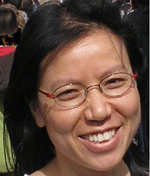# Faculty - Dr. Dilian Yang### Dr. Dilian Yang, Ph.D. (Waterloo)

Professor
dyang@uwindsor.ca
(519) 253-3000 x3042
Lambton Tower 9-116
Research Area: Operator Algebras and Functional Equations

#### Research Interests

My main research interests are operator algebras and functional equations.

On operator algebras, I am currently interested in higher rank graph algebras. They are natural operator algebras associated with higher rank graphs, which are higher dimensional analogues of directed graphs. They have been attracting a great deal of attention recently. On one hand, there are some results generalized from graph algebras; on the other hand, they are significantly more complicated than graph algebras, and provide more intriguing operator algebras.
On functional equations, I am interested in studying them by applying harmonic analysis and representation theory. This gives close connections between functional equations and other areas.

#### Editioral Board

• I am an editor for Banach Journal of Mathematical Analysis (2014 - )

#### Recent Publications

##### Operator Algebras:
• H. Li and D. Yang (2017), Boundary quotient C*-algebras of products of odometers. Canadian Journal of Mathematics, 32 pages, to appear, 2017. http://dx.doi.org/10.4153/CJM-2017-034-5.
• J. Brown, H. Li and D. Yang (2017), Cartan subalgebras of topological graph algebras and k-graph C*- algebras, Munster Journal of Mathematics, 14 pages, to appear, 2017. https://www.uni-muenster.de/FB10/mjm/acc.html.
• D. Yang, the interplay between k-graphs and the Yang-Baxter equation, Journal of Algebra 451 (2016), 494-525.
• D. Yang, Cycline subalgebras of k-graph C*-algebras, Proceedings of the American Mathematical Society 144 (2016), 2959-2969.
• D. Yang, Factoriality and type classification of k-graph von Neumann algebras, Proceedings of the Edinburgh Mathematical Society 60 (2017), 499-518.
• D. Yang, Analytic free semigroup algebras and Hopf algebras, Houston J. Math. 42 (2016), 919-943.
• D. Yang, Periodic k-graph algebras revisited, J. Aust. Math. Soc. 99 (2015), 267-286.
• A. Fuller and D. Yang, Nonself-adjoint 2-graph algebras, Transactions of the America Mathematical Society 367 (2015), 6199-6224.
• M. Kennedy and D. Yang, A non-self-adjoint Lebesgue decomposition, Analysis & PDE 7(2014), 497-512.
• M. Kennedy and D. Yang, The Hopf structure of some dual algebras, Integral Equations and Operator Theory 79 (2014), 191-217.
• D. Yang, Type III Von Neumann algebras associated with 2-graph, Bulletin of the London Mathematical Society 44 (2012), 675-686.
• D. Yang, Endomorphisms and modular theory of 2-graph C*-algebras, Indiana Univ. Math. J. 59 (2010) 495-520.
• K. R. Davidson, S. C. Power and D. Yang, Dilation theory for rank 2 graph algebras, J. Operator Theory 63 (2010), 245-270.
• K. R. Davidson and D. Yang, Representations of higher rank graph algebras, New York J. Math. 15 (2009), 169–198.
• K. R. Davidson and D. Yang, Periodicity in rank 2 graph algebras, Canad. J. Math. 61 (2009), 1239–1261.
• K. R. Davidson, S. C. Power and D. Yang, Atomic representations of rank 2 graph algebras, J. Funct. Anal. 255 (2008), 819–853.
• K. R. Davidson and D. Yang, A note on absolute continuity in free semigroup algebras, Houston J. Math. 34 (2008), 283–288.
##### Functional Equations:
• D. Ilisevi, A. Turnsek and D. Yang, Orthogonally Additive Mappings on Hilbert Modules, Studia Mathematica 221 (2014), 209-229.
• D. Yang, Functional equations and Fourier analysis, Canadian Mathematical Bulletin 56 (2013), 218-224.
• J. An and D. Yang, Nonabelian harmonic analysis and functional equations on compact groups, J. Lie Theory 21 (2011), 427-456.
• D. Yang, The symmetrized sine additional formula, Aequationes Math. 82 (2011), 299-318.
• J. An and D. Yang, ALevi-Civita equation on compact groups and nonabelian Fourier analysis,  Integral Equations Operator Theory 66 (2010), 183-195.
• L. Li, D. Yang and W. Zhang, A note on iterative roots of PM functions, J. Math. Anal. Appl. 341 (2008), 1482–1486.
• D. Yang, Factorization of cosine functions on compact connected groups, Math. Z. 254 (2006), 655–674.
• D. Yang, Orthogonality spaces and orthogonally additive mappings, Acta. Math. Hungar. 113 (2006), 269-280.